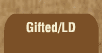Mathematics and Dyslexia

Tips for Learning the Times Tables

Tools for the Times Tables & Workbook

Home > Math > times tables practice > Eights big (in order)

## The Eight Times Tables In Order - second set

You should know 8 x 6, 8 x 7, 8 x 9, and 8 x 10 already -- the only one left is 8 x 8.

Practice this page until they are easy and fast... and if the other ones still are, then ... you know your times tables.

1. 8 x 6=
2. 8 x 7=
3. 8 x 8=
4. 8 x 9=
5. 8 x 10=
6. 10 x 8=
7. 9 x 8=
8. 8 x 8=
9. 7 x 8=
10. 6 x 8=
11. 8 x 8=
12. 7 x 8=
13. 9 x 8=
14. 8 x 8=
15. 8 x 7=